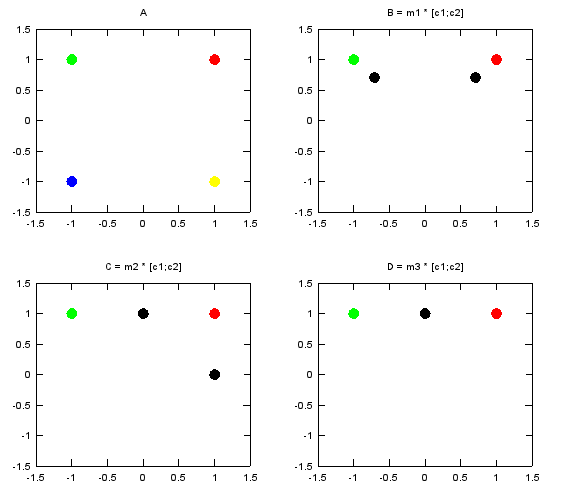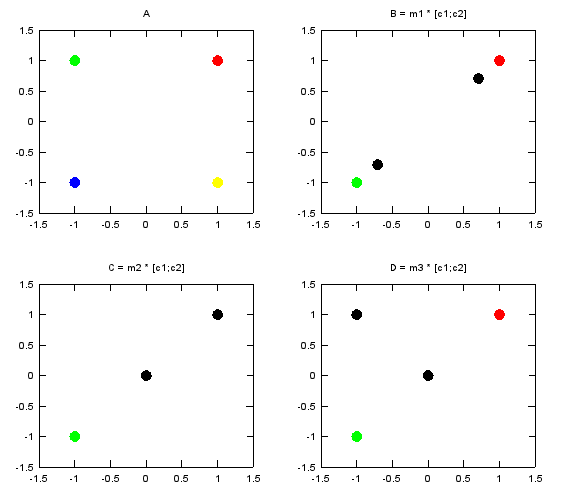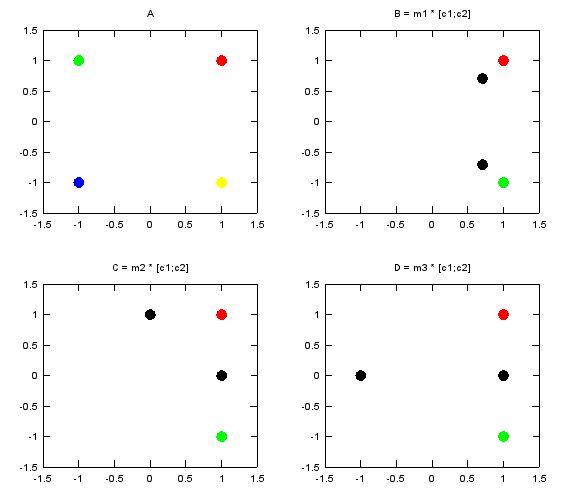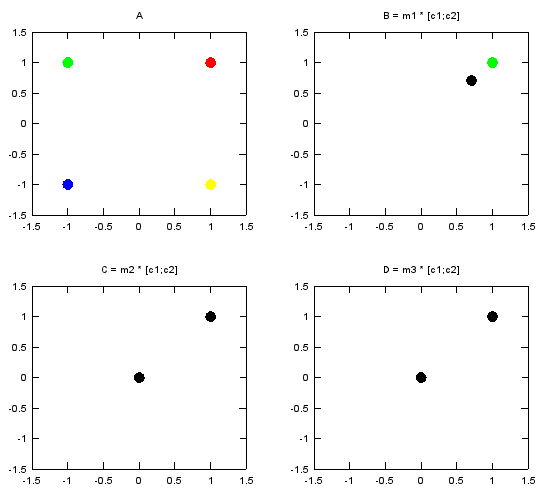Engineering Math - Quick Reference                                 Home : www.sharetechnote.com Matrix-of Complex Numbers   In the above section, you saw some examples of how a matrix can transform a shape in a coordinate. But all the elements in the matrix and the numbers representing a point in a coordinate were real numbers. In this section,  you would see examples where complex numbers are used in both matrix and coordinate. As you may see in complex number section, the operation of the complex number itself has some geometric transformation properties. Therefore, the final outcome of the transformation of complex coordinate and complex matrix are even more complicated. Only real practice on your own would give you the real meaning of those transformation.   This kind of transformation are used pretty often in MIMO (Multiple Input Multiple Output) in wireless communication.   Let's directly jump into some examples. Following is a Matlab/Octave code that I wrote. v1, v2, v3, v4 are a complex number representing a constellation point in I/Q coordinate. m1,m2,m3 is a complex transformation matrix. c1, c2 can be any two complex numbers selected from {v1, v2, v3, v4}. Just try this code and observe the result. Change any of v1,v2,v3,v4, c1,c2, m1,m2,m3 and rerun the code. Repeat this process until your brain get the overall pictures of how this transformation work.   v1 = 1 + j; v2 = -1 + j; v3 = -1 - j; v4 = 1 - j;   c1 = v1; c2 = v2;   m1 = 1/sqrt(2).*[1 0; 0 1] m2 = 1/2.*[1 1; 1 -1] m3 = 1/2.*[1 1; j -j]   m1_c12 = m1 * [c1;c2] m2_c12 = m2 * [c1;c2] m3_c12 = m3 * [c1;c2]   subplot(2,2,1); plot(real(v1), imag(v1),'ro','MarkerFaceColor',[1 0 0], 'MarkerSize',10, ...      real(v2), imag(v2),'go','MarkerFaceColor',[0 1 0], 'MarkerSize',10, ...      real(v3), imag(v3),'bo','MarkerFaceColor',[0 0 1], 'MarkerSize',10, ...      real(v4), imag(v4),'yo','MarkerFaceColor',[1 1 0], 'MarkerSize',10);      axis([-1.5 1.5 -1.5 1.5]);      title('A');      subplot(2,2,2); plot(real(c1), imag(c1),'ro','MarkerFaceColor',[1 0 0], 'MarkerSize',10, ...      real(c2), imag(c2),'go','MarkerFaceColor',[0 1 0], 'MarkerSize',10, ...      real(m1_c12), imag(m1_c12),'ko','MarkerFaceColor',[0 0 0], 'MarkerSize',10);      axis([-1.5 1.5 -1.5 1.5]);      title('B = m1 * [c1;c2]');   subplot(2,2,3); plot(real(c1), imag(c1),'ro','MarkerFaceColor',[1 0 0], 'MarkerSize',10, ...      real(c2), imag(c2),'go','MarkerFaceColor',[0 1 0], 'MarkerSize',10, ...      real(m2_c12), imag(m2_c12),'ko','MarkerFaceColor',[0 0 0], 'MarkerSize',10);      axis([-1.5 1.5 -1.5 1.5]);      title('C = m2 * [c1;c2]');   subplot(2,2,4); plot(real(c1), imag(c1),'ro','MarkerFaceColor',[1 0 0], 'MarkerSize',10, ...      real(c2), imag(c2),'go','MarkerFaceColor',[0 1 0], 'MarkerSize',10, ...      real(m3_c12), imag(m3_c12),'ko','MarkerFaceColor',[0 0 0], 'MarkerSize',10);      axis([-1.5 1.5 -1.5 1.5]);      title('D = m3 * [c1;c2]');   As the first example, I selected v1 and v2 as the two numbers to be transformed by the matrix. Following is the numerical result of the transformation. m1_c12 is the result of transformation of (c1,c2) by the matrix m1. m2_c12 is the result of transformation of (c1,c2) by the matrix m2. m3_c12 is the result of transformation of (c1,c2) by the matrix m3.   c1 = v1; c2 = v2; m1_c12 =      0.70711 + 0.70711i   -0.70711 + 0.70711i   m2_c12 =      0 + 1i    1 + 0i   m3_c12 =      0 + 1i    0 + 1i   Following is the graphical representation of the result of this transformation. Graph A shows the four complex numbers v1,v2,v3,v4 in I/Q coordinate. Red = v1 Green = v2 Blue = v3 Yellow = v4 Black = the result of transformation of (c1,c2) by m1, m2, m3In the second example, I selected v1 and v3 as the two numbers to be transformed by the matrix. Following is the numerical result of the transformation. m1_c12 is the result of transformation of (c1,c2) by the matrix m1. m2_c12 is the result of transformation of (c1,c2) by the matrix m2. m3_c12 is the result of transformation of (c1,c2) by the matrix m3.   c1 = v1; c2 = v3;   m1_c12 =      0.70711 + 0.70711i   -0.70711 - 0.70711i   m2_c12 =      0 + 0i    1 + 1i   m3_c12 =      0 + 0i   -1 + 1i   Following is the graphical representation of the result of this transformation. Graph A shows the four complex numbers v1,v2,v3,v4 in I/Q coordinate. Red = v1 Green = v2 Blue = v3 Yellow = v4 Black = the result of transformation of (c1,c2) by m1, m2, m3In the third example, I selected v1 and v4 as the two numbers to be transformed by the matrix. Following is the numerical result of the transformation. m1_c12 is the result of transformation of (c1,c2) by the matrix m1. m2_c12 is the result of transformation of (c1,c2) by the matrix m2. m3_c12 is the result of transformation of (c1,c2) by the matrix m3.   c1 = v1; c2 = v4;   m1_c12 =      0.70711 + 0.70711i    0.70711 - 0.70711i   m2_c12 =      1 + 0i    0 + 1i   m3_c12 =      1   -1   Following is the graphical representation of the result of this transformation. Graph A shows the four complex numbers v1,v2,v3,v4 in I/Q coordinate. Red = v1 Green = v2 Blue = v3 Yellow = v4 Black = the result of transformation of (c1,c2) by m1, m2, m3In the fourth example, I selected v1 and v1 again as the two numbers to be transformed by the matrix. Following is the numerical result of the transformation. m1_c12 is the result of transformation of (c1,c2) by the matrix m1. m2_c12 is the result of transformation of (c1,c2) by the matrix m2. m3_c12 is the result of transformation of (c1,c2) by the matrix m3.   c1 = v1; c2 = v1;   m1_c12 =      0.70711 + 0.70711i    0.70711 + 0.70711i   m2_c12 =      1 + 1i    0 + 0i   m3_c12 =      1 + 1i    0 + 0i   Following is the graphical representation of the result of this transformation. Graph A shows the four complex numbers v1,v2,v3,v4 in I/Q coordinate. Red = v1 Green = v2 Blue = v3 Yellow = v4 Black = the result of transformation of (c1,c2) by m1, m2, m3